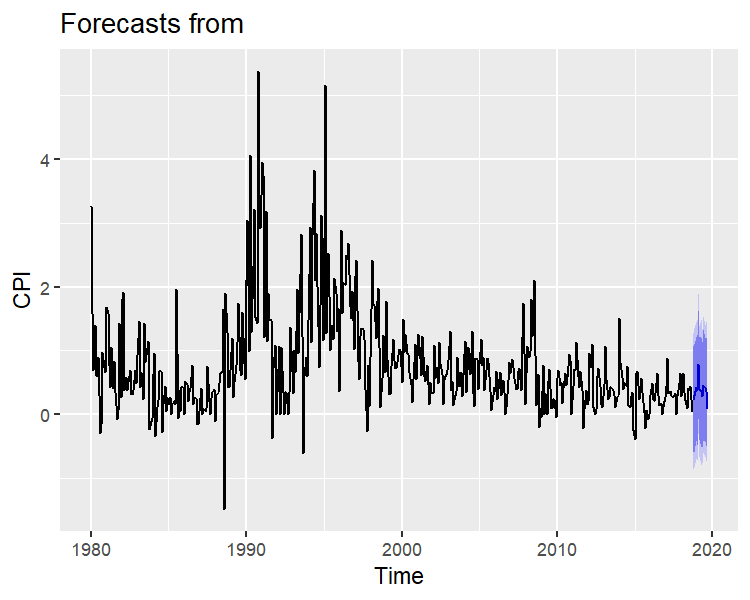# Estimating ARIMA Models

## Introduction

The core inflation index is used to measure aggregate demand pressures that the action of monetary policy can modify; it is a partial measure derived from inflation calculated through the Consumer Price Index (CPI). This vignette explains how to estimate a ARIMA model to predict the CPI in Honduras for the next years using the functions check_residuals and stan_sarima in the bayesforecast package.

Here we will show how to carry out a few parts of the analysis from Chapter 8.7 of Hyndman & Khandakar, 2008 that is:

1. Plot the data and identify any unusual observations.

2. Choose a model and check the residuals from your chosen model by plotting the ACF of the residuals.

3. If residuals look like white noise, we calculate the forecasts; otherwise, we choose another model, an adaptation of the Hyndman process and not the whole process as such since, in Bayesian statistics, the AIC and BIC are not commonly used.

Graphing the data:

autoplot(object = ipc,main = "Inflation rate in Honduras",ylab="CPI")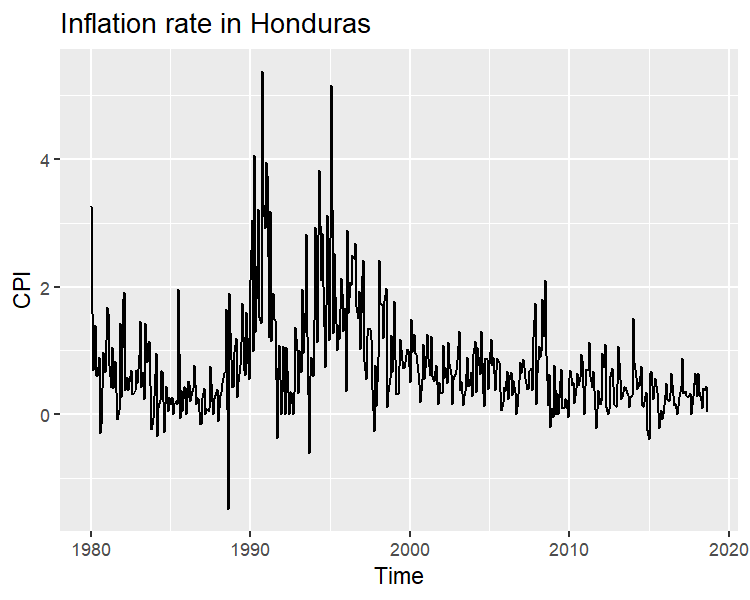The previous figure shows that the series does not seem to follow a stationary behavior and has some asymmetry, then the series will be differentiated, and we will graph the correlation graphs.

g1 = autoplot(object = diff(ipc),main = "Differentiated series on inflation in Honduras",y = "CPI")
g2 = ggacf(y = diff(ipc))
g3 = ggpacf(y = diff(ipc))

gridExtra::grid.arrange(g1,g2,g3,
layout_matrix = matrix(c(1,2,1,3),nrow = 2))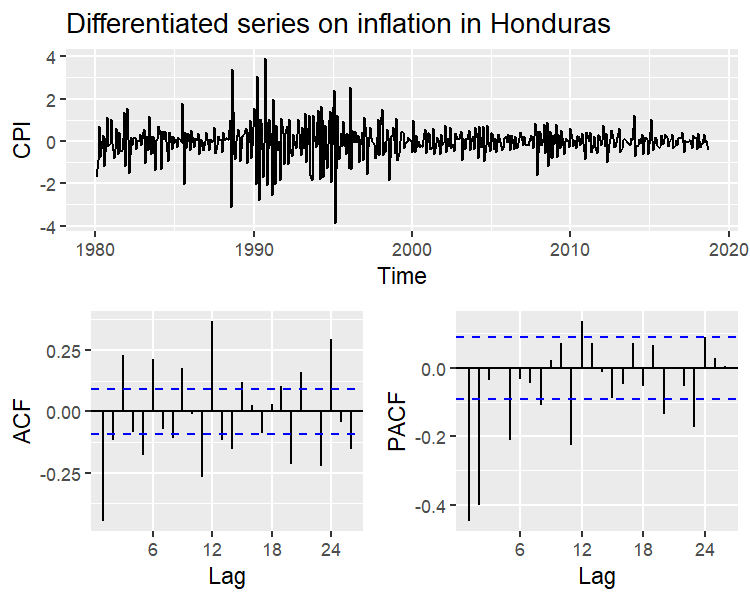The autocorrelation functions (ACF) and partial autocorrelation (PACF) show a mild correlation of at most a data lag, then the order of the ARIMA Model to be considered is p = 1, d = 1, q = 1, additionally both graphs show weak periodic patterns. Hence the order of the seasonal component is P = 1, D = 0, Q = 1. We consider weakly informative prior distributions, using the priors by defect for the location, scale, autoregressive, and moving average parameters. For the seasonals components, we will use a beta(2,2) distribution with domain $$D = [1,-1]$$. As a result, the complete model is:

$Inflacion \sim SARIMA(1,1,1)_\times(1,0,1)\\ \mu_0 \sim t(0,2.5,7)\\ \sigma_0 \sim t(7)\\ ar_1, ma_1 \sim N(0,0.5)\\ sar_1,sma_1 \sim beta(2,2)$

bayesforecast offers a similar interface to the rstanarm and forecast packages, the model can be estimated using the following instructions:

sf1 = stan_sarima(ts = ipc,order = c(1,1,1),seasonal = c(1,1,1),
prior_sar = beta(2,2),prior_sma = beta(2,2),chains = 1)

summary(sf1)
#>             mean     se        5%       95%      ess   Rhat
#> mu0       0.0032 0.0002   -0.0078    0.0142 920.8685 1.0001
#> sigma0    0.6452 0.0007    0.6096    0.6822 941.3985 1.0024
#> ar       -0.1919 0.0033   -0.3899   -0.0437 883.2747 1.0042
#> ma       -0.4338 0.0049   -0.6128   -0.0933 945.8398 1.0020
#> sar      -0.2616 0.0032   -0.3877   -0.0646 996.8215 0.9993
#> sma      -0.3511 0.0041   -0.6743   -0.2041 841.9779 1.0017
#> loglik -443.0468 0.0582 -446.5720 -440.6317 784.8410 1.0000

To check the adjustment of each parameter, we review the chains and density graphs of each model:

mcmc_plot(object = sf1)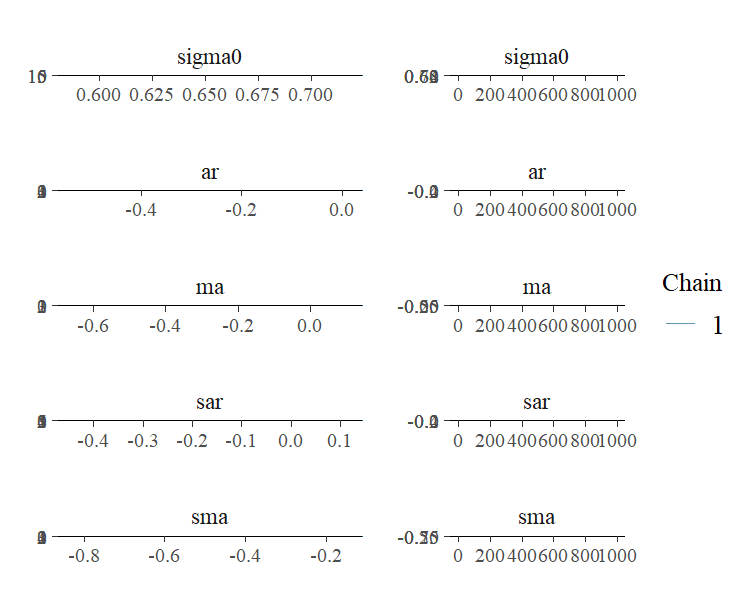The chains seem to converge and not observed multimodality in the parameter distributions. Therefore, we consider accepting the estimation of the parameters; we proceed to review the fit.

autoplot(sf1)+labs(title = "Posterior Predict", y="CPI")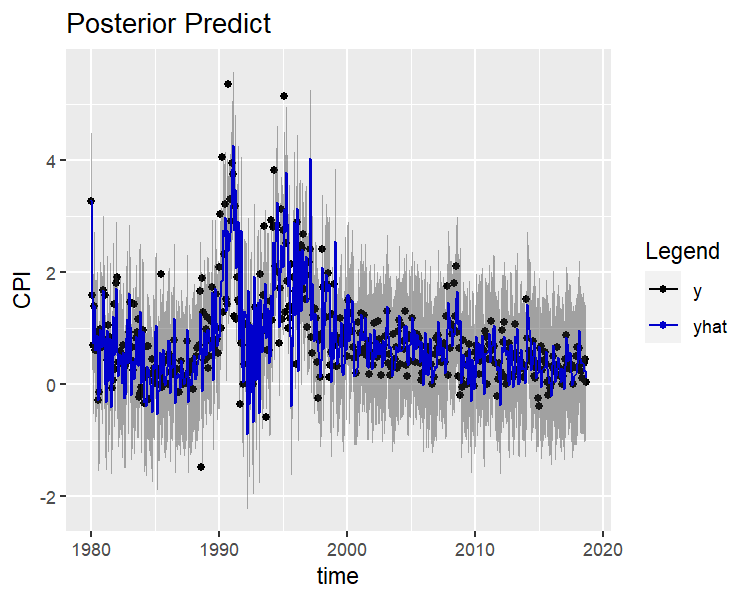The model seems to fit the series, we proceed to review the model residuals, the check_residuals function estimates the posterior mean of the residuals and plots them. This plot is not sufficient to corroborate the assumptions of normality and stationarity but is an initial indicator of the adjustment.

check_residuals(sf1)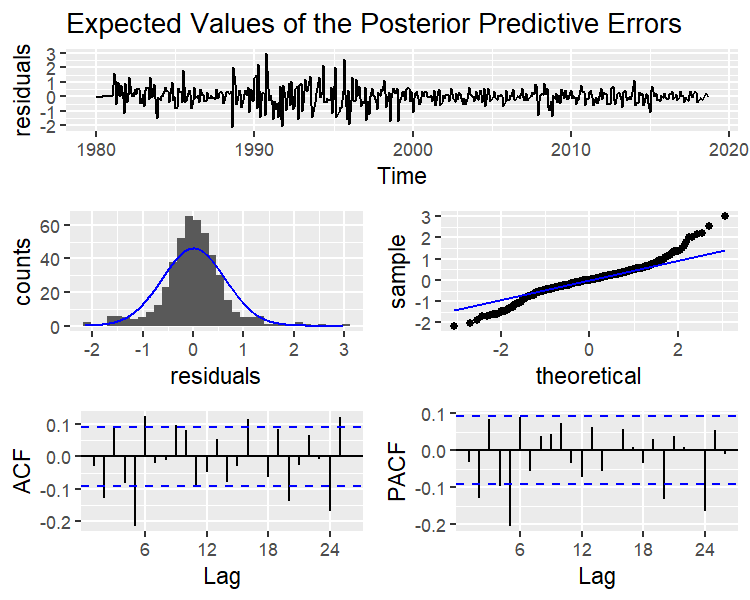The series of residuals (upper part) shows that the model does not explain the period between 1900-2000 due to the intense volatility present in that decade. The histogram and quantile graph (middle part) show that the model has heavy tails due to the high volatility of the series. Finally, the residuals’ auto-correlation presents a certain periodicity. Therefore, a seasonal period was not enough to model the seasonal pattern.

## Forecast

Finally, we predict the model for the next year:

autoplot(object = forecast(sf1,h = 12),ylab="CPI")